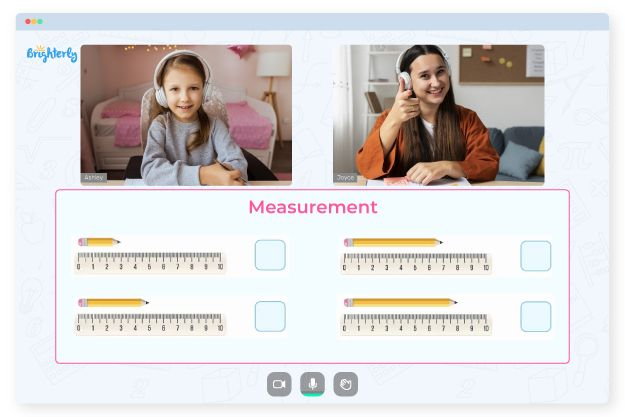# Measuring Lengths Worksheets

Length is a fundamental concept in geometry and can be defined as the distance between two points. Various measuring length worksheets pdf can help students learn how to measure lengths. These worksheets typically include activities and exercises designed to help students understand the concept of measuring lengths and develop their skills in using rulers and other measuring tools.

## How It Works

By using measuring length worksheets, children will need a measuring tool such as a ruler, tape measure, or yardstick to measure length. They will place the beginning of the measuring instrument at one end of the object or distance you want to measure and then extend the device along the length of the thing until the other end is reached. If they use a standard ruler, the length is indicated in inches or fractions of an inch. The distance is marked in feet and inches if they use a tape measure or yardstick.

Math for Kids

Is Your Child Struggling With Math?
1:1 Online Math Tutoring## Benefits of Measuring Lengths Worksheets

Kids can use the arc length and radian measure worksheet answer key to understand and compare the sizes of different objects or distances, which can be helpful in different ways. In addition, the measuring length worksheet answer key teaches kids significant mathematical concepts that are essential to understanding geometry, algebra, and other math subjects.### Measuring Lengths Worksheets PDF

Measuring Length Worksheets### Measuring Lengths Worksheets PDF

Measuring Length Worksheets PDF### Measuring Lengths Worksheets PDF### More Measurement Worksheets

Struggling with Measurement?• Is your child having a hard time understanding measurement concepts?
• An online tutor could be the solution.

Does your child need extra help with measurement concepts? Try studying with an online tutor.